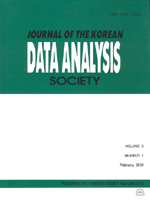상세검색
최근 검색어 전체 삭제
다국어입력
즐겨찾기0KCI등재 학술저널

# Threshold Models for Count Time Series: Case Study

• 등재여부 : KCI등재
• 2016.02
• 9 - 18 (10 pages)
• 2

This article briefly summarizes the self-exciting threshold integer-valued autoregressive model with 2 regimes of order 1 (SETINAR(2,1)) model and the self-excited threshold Poisson autoregression (SETPAR) model. For SEPAR model, it is assumed that the observations follow a Poisson distribution conditioned on an accompanying intensity process, which is equipped with a two-regime structure according to the magnitude of the lagged observations. The SEPAR model allows for more general modelling framework for the intensity process, including the negative serial dependence in the data, which is differentiated from Poisson integer-valued GARCH (INGARCH) model. The SERINAR(2,1) model uses the binomial binomial thinning &#8728; operation, which was introduced by Steutel, van Harn (1979) to adapt the term of self-decomposability and stability for integer-valued time series. The SETINAR(2,1) model also assumes a two regime structure of process according to the magnitude of the lagged observations. Theses two models are very recently introduced for time series of counts, respectively 2012, 2014. A real data application are considered, where the models are applied to the number of contract strikes in U.S. manufacturing from 1968 to 1976.

1. Introduction

2. Threshold autoregressive models

3. Threshold models for count time series

4. Real data analysis

5. Concluding remarks

References